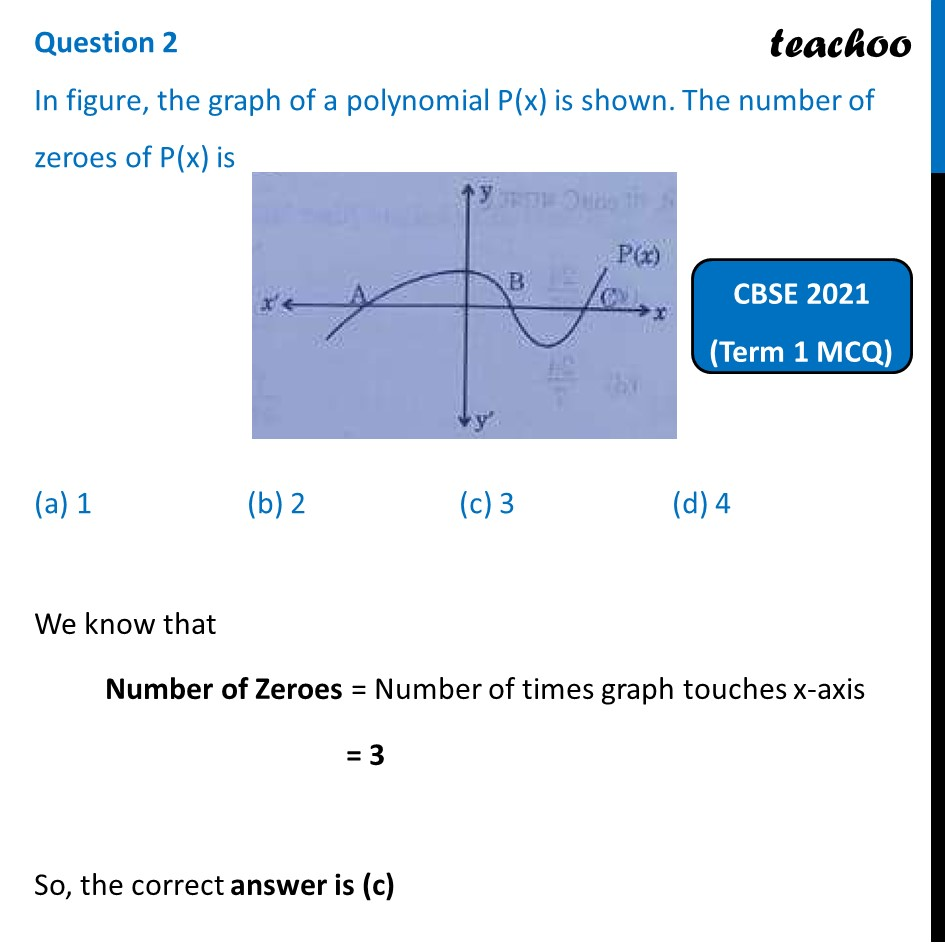Past Year MCQ (Maths Standard)

Chapter 2 Class 10 Polynomials
Serial order wise

## (d) 4

This question is similar from Example 1 - Chapter 2 Class 10 - PolynomialsGet live Maths 1-on-1 Classs - Class 6 to 12

### Transcript

Question 2 In figure, the graph of a polynomial P(x) is shown. The number of zeroes of P(x) is (a) 1 (b) 2 (c) 3 (d) 4 We know that Number of Zeroes = Number of times graph touches x-axis = 3 So, the correct answer is (c)